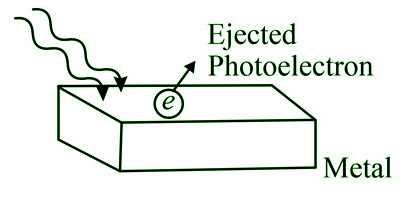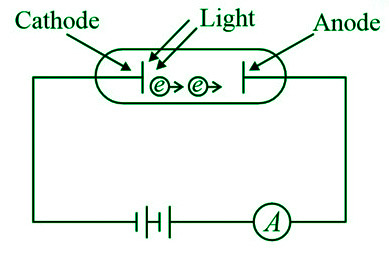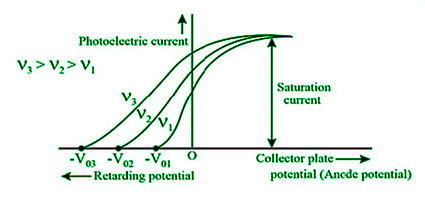GeeksforGeeks App
Open AppBrowser
Continue

# Photoelectric Effect – Definition, Equation, Characteristics, Applications

The dual nature of matter and the dual nature of radiation were groundbreaking physics notions. Scientists discovered one of nature’s best-kept secrets around the turn of the twentieth century: wave-particle duality, or the dual nature of matter and radiation. Everything is made up of waves and particles.

### What is a Photon?

A photon is the smallest discrete amount of electromagnetic energy, also known as a quantum. It’s the fundamental unit of all light.

Photons are continually in motion and travel at a constant speed of 2.998 x 108 m/s to all observers in a vacuum. The speed of light, indicated by the letter c. Every photon has a specific quantity of energy and momentum. The photon’s energy is provided by,

E=hν

where,

• h is the Planck’s constant. The value of the Planck constant is h=6.626×10–34 J s
• v is the frequency of the light.

The momentum of a photon is given by,

p=h/λ

where,

• λ is the wavelength of light.
• h is the Planck’s constant.

Properties of Photon

Photons have the following basic properties:

• The quantity of photons crossing an area per unit time increases as light intensity increases. It has no effect on the radiation’s energy.
• Electric and magnetic fields have no effect on a photon. It has no electrical charge.
• A photon is massless.
• It’s a sturdily constructed particle.
• When radiation is emitted or absorbed, photons can be generated or destroyed.
• During a photon-electron collision, the whole energy and momentum are conserved.
• A photon is incapable of decay on its own.
• A photon’s energy can be transferred when it interacts with other particles.
• In contrast to electrons, which have a spin of 1/2, a photon has a spin of one. Its spin axis is perpendicular to the travel direction. The polarization of light is supported by this feature of photons.

### Photoelectric Effect

When a metal is exposed to light, the photoelectric effect occurs, in which the metal emits electrons from its valence shell.  The emitted electron is known as photoelectron, and this phenomenon is commonly known as photoemission.

Wilhelm Ludwig Franz Hallwachs was the first to notice the photoelectric effect, which Heinrich Rudolf Hertz later confirmed. This phenomenon, as well as the quantum nature of light, were explained by Einstein. In 1921, Einstein was awarded the Nobel Prize for Physics for his work on the Photoelectric Effect.Threshold Energy for the Photoelectric Effect

The photons that strike the metal’s surface must have enough energy to overcome the attractive forces that bind the electrons to the nuclei in order for the photoelectric effect to occur. The threshold energy (represented by the symbol Φ) is the least amount of energy required to remove an electron from a metal. A photon’s frequency must be identical to the threshold frequency in order for it to have the same energy as the threshold energy (which is the minimum frequency of light required for the photoelectric effect to occur). The corresponding wavelength (called the threshold wavelength) is generally denoted by the sign λth, and the threshold frequency is usually denoted by the symbol νth. The following is the link between the threshold energy and the threshold frequency.

Φ = hνth = hc / λth

Relationship between the Frequency of the Incident Photon and the Kinetic Energy of the Emitted Photoelectron

Ephoton = Φ + Eelectron

hν = hνth + 1/2 mev2

where,

• Ephoton signifies the incident photon’s energy, which is equal to hν.
• Φ signifies the metal surface’s threshold energy, which is equal to hνth.
• Eelectron is the photoelectron’s kinetic energy, which is 1/2mev2 (me = mass of electron = 9.1 x 10-31 kg).

There will be no emission of photoelectrons if the photon’s energy is less than the threshold energy (since the attractive forces between the nuclei and the electrons cannot be overcome). As a result, if ν < νth , the photoelectric effect will not occur. There will be an emission of photoelectrons if the photon frequency is exactly equal to the threshold frequency (ν=νth), but their kinetic energy will be zero.

### Minimum Condition for Photoelectric Effect

• Threshold Frequency (γth): The threshold frequency for the metal is the lowest frequency of incident light or radiation that will generate a photoelectric effect, i.e. ejection of photoelectrons from a metal surface. It is constant for one metal, but various metals may have varying values.

If γ = frequency of incident photon and γth= threshold frequency, then,

1. If γ < γth, there will be no photoelectron ejection and, as a result, no photoelectric effect.
2. If γ=γth, photoelectrons are simply expelled from the metal surface, and the electron’s kinetic energy is zero.
3. If γ>γth, photoelectrons, and kinetic energy will be ejected from the surface.
• Threshold Wavelength (λth): The metal surface with the largest wavelength to incident light is known as the threshold wavelength during electron emission.

λth = c/γth

For λ = wavelength of the incident photon, then

1. If λ<λth, the photoelectric effect will occur, and the expelled electron will have kinetic energy.
2. If λ= λth, the photoelectric effect will be the only one that occurs, and the kinetic energy of the ejected photoelectron will be zero.
3. There will be no photoelectric effect if λ>λth.
• Work Function or Threshold Energy (Φ): The work function/threshold energy is the minimum amount of thermodynamic work required to remove an electron from a conductor to a location in the vacuum just outside the conductor’s surface.

Φ = hγth = hc/λth

If E = energy of an incident photon, then

1. If E<Φ, there will be no photoelectric effect.
2. If E =Φ, just the photoelectric effect occurs, but the kinetic energy of the expelled photoelectron is 0.
3. If E > photoelectron, photoelectron will be zero.
4. If E >Φ, the photoelectric effect will occur, as will the expelled electron’s possession of the kinetic energy.

Principle of Photoelectric Effect: A metal surface is irradiated with light in the photoelectric effect, and when light falls on the metal’s surface, photoemission occurs, and photoelectrons are ejected from the metal’s surface. The energy of the wave’s photon is transmitted to the metal atom’s electrons, which causes the electrons to get excited and expelled with a certain velocity.

### Equation of Photoelectric Effect

The photon’s energy is equal to the sum of the metal’s threshold energy and the photoelectron’s kinetic energy.Thus, the equation of photoelectric wave is given by,

KEmax=hv–ϕ

where,

• KEmax is the maximum kinetic energy of the photoelectron
• hv is the energy of the photon.
• φ is the work function of the metal

Work function is determined by the metal in question, and it will change if the metal is changed. The work function is sometimes defined in terms of threshold frequency, which is the frequency of light for which the emitted Photoelectron’s maximal kinetic energy is zero.

ϕ=hv0

where,

• v0 is the threshold frequency.
• h is the Planck’s constant.

The maximum kinetic energy remains constant as the light intensity increases, but the value of photocurrent increases.Characteristics Of Photoelectric Effect

• The threshold frequency varies by material; different materials have varying threshold frequencies.
• The photoelectric current is proportional to the intensity of light.
• The photoelectrons’ kinetic energy is related to the frequency of light.
• The frequency is directly proportional to the stopping potential, and the process is immediate.

Factors affecting Photoelectric Effect

The photoelectric effect depends on :

1. The intensity of incident radiation.
2. A potential difference between metal plate and collector.

### Applications of Photoelectric Effect

• Solar Panels use it to generate power. Metal combinations in these panels allow power to be generated from a wide variety of wavelengths.
• Sensors for motion and position: A photoelectric material is placed in front of a UV or IR LED in this case. Light is switched off when an object is placed between the LED and the sensor, and the electronic circuit recognizes a change in potential difference.
• Lighting sensors, such as those found in smartphones, allow for automatic screen brightness adjustment in response to ambient light. This is due to the fact that the quantity currently created by the photoelectric effect is proportional to the amount of light that strikes the sensor.
• Digital cameras can detect and record light because they have photoelectric sensors that respond to different colours of light.
• X-Ray Photoelectron Spectroscopy (XPS): This approach involves irradiating a surface with x-rays and measuring the kinetic energy of the electrons that are released. Important features of a surface’s chemistry, such as elemental composition, chemical composition, the empirical formula of compounds, and chemical state, can be acquired.
• In burglar alarms, photoelectric cells are utilized.
• Photomultipliers use it to detect low light levels.
• In the early days of television, it was used in video camera tubes.
• This phenomenon is used in night vision systems.
• The photoelectric effect is also useful in the research of nuclear reactions. Because released electrons tend to carry specific energy that is distinctive of the atomic source, it is used in the chemical study of materials.

### Sample Questions

Question 1: What is the mass of a photon?

The photon’s rest mass is zero, which indicates that if the photon is moving, it will have some momentum, which is equivalent to mass, but at rest, the photon’s mass will be zero.

Question 2: What is threshold frequency?

The threshold frequency of light is the frequency at which the photoelectron’s kinetic energy is zero and it is just enough to emit photoelectron. The work function of the metal is equal to the energy associated with threshold frequency.

Question 3: Light of wavelength 4000Å is incident on a metal plate whose work function is 2eV. What is the maximum kinetic energy of emitted photoelectron?

The wavelength of light is λ=4000Å and work function, φ0=2eV

From the Einstein Photoelectric equation, the maximum kinetic energy of photoelectron is given by,

Kmax=(hc/λ–φ0)

where ‘h′ is Planck’s constant and ‘c′ is the speed of light in a vacuum.

Kmax=(6.6×10–34×3×108/4000×10–10–(2×1.6×10–19))

Kmax=4.95×10–19/1.6×10–19eV–2eV=1.1eV

The maximum kinetic energy 1.1eV.

Question 4: The value of retarding potential needed to stop the photoelectrons ejected from a metal surface of work function 1.2eV with the light of energy 2eV is

Work function of the metalφ=1.2eV and energy of the photons is hν=2eV.

The maximal kinetic energy of photoelectrons is given by the Einstein photoelectric equation:

eV=hv–φ

Where ‘V′ is retarding potential or stopping potential.

h is the Planck’s constant.

φ is the work function of the metal.

V=(2eV–12eV)/e=0.8V

Thus, the retarding potential is 0.8V

Question 5: What is stopping potential?